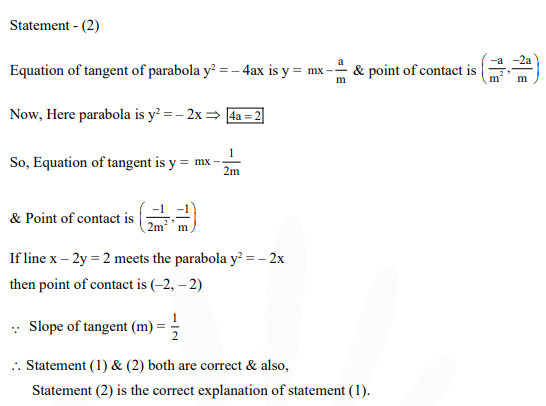# Statement 1 : The line

Question:

Statement 1 : The line $\mathrm{x}-2 \mathrm{y}=2$ meets the parabola, $\mathrm{y}^{2}+2 \mathrm{x}=0$ only at the point $(-2,-2)$

Statement 2 : The line $\mathrm{y}=\mathrm{mx}-\frac{1}{2 \mathrm{~m}}\left(\mathrm{~m}^{1} 0\right)$ is tangent to the parabola, $\mathrm{y}^{2}=-2 \mathrm{x}$ at the point $\left(-\frac{1}{2 m^{2}},-\frac{1}{m}\right)$

1. Statement 1 is false; Statement 2 is true.

2. Statement l is true; Statement 2 is true; Statement 2 is not a correct explanation for Statement1.

3. Statement 1 is true; Statement 2 is false.

4. Statement 1 is true; Statement 2 is true; Statement 2 is a correct explanation for Statement1.

Correct Option:

Solution: